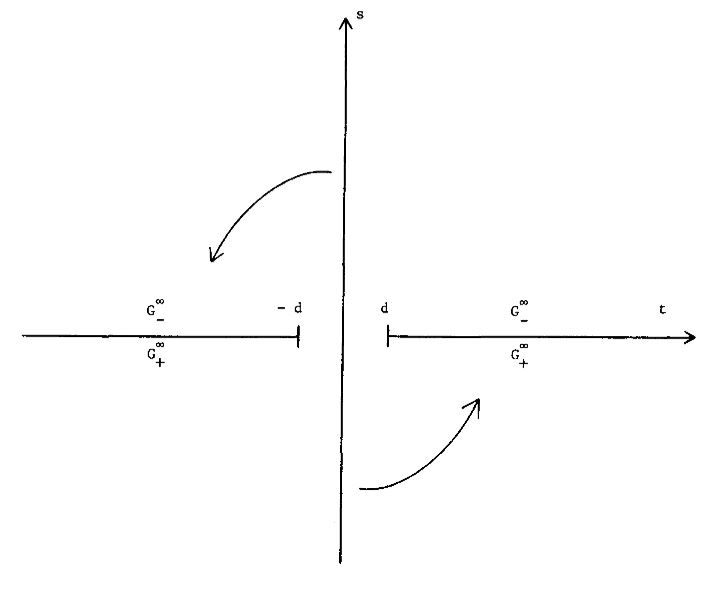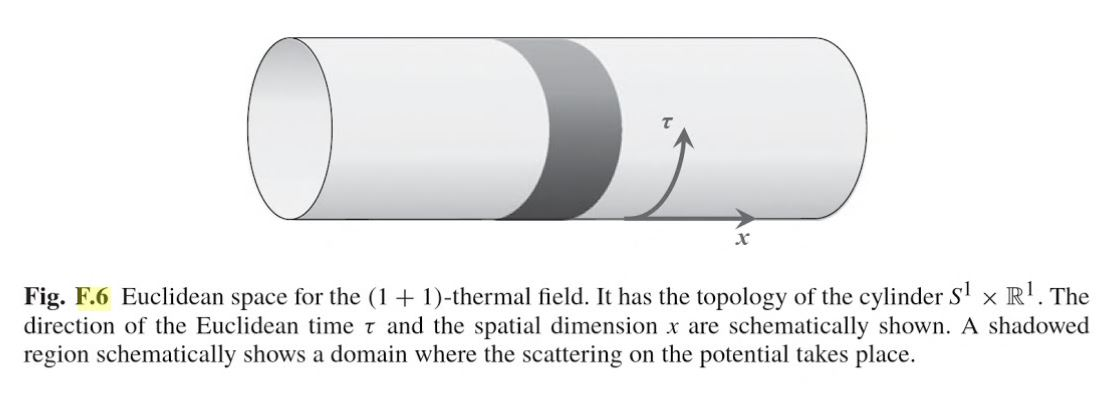Contents

# Contents

## Idea

What is called Wick rotation (after Gian-Carlo Wick) is a method in physics for finding a construction in relativistic field theory on Minkowski spacetime or, more generally, on Lorentzian manifolds, from a related construction in Euclidean field theory on Riemannian manifolds in a way that involves or generalizes the idea of analytic continuation for the time coordinates.The complex plane for a complexified time coordinate $t + i s$. After Wick rotation it is the imaginary part $s$ that replaces the “real” time $t$.

graphics grabbed from Fulling-Ruijsenaars 87

This is motivated by the observation that the Minkowski metric (with the $-1,1,1,1$ convention) and the four-Euclidean metric are equivalent if the time components of either are allowed to have imaginary values. Hence Wick rotation, when it applies, involves analytic continuation of n-point functions to complex valued time coordinates.

For relativistic/Euclidean quantum field theory on Minkowski spacetime $\mathbb{R}^{d,1}$/Euclidean space $\mathbb{R}^{d+1}$ Wick rotation is rigorously understood and takes the form of a theorem relating the Wightman axioms for n-point functions in relativistic field theory to the Osterwalder-Schrader axioms for correlators in Euclidean field theory.

See for instance Fulling-Ruijsenaars 87, section 2 for a clear account.

More generally, in this context Wick rotation applies to relativistic field theory in thermal equilibrium states at positive temperature $T$ (“KMS states”), and then relates this to Euclidean field theory with compact/periodic Euclidean time of length $\beta = 1/T$ (see Fulling-Ruijsenaars 87, section 3):

$\array{ \left. \array{ \text{relativistic field theory} \\ \text{on Minkowski spacetime} \\ \mathbb{R}^{d,1} \\ \text{in a thermal equilibrium state} \\ \text{at temperature}\; T } \right\} & \;\;\;\; \overset{ \text{Wick rotation} }{\leftrightarrow} \;\;\;\; & \left\{ \array{ \text{Euclidean field theory} \\ \text{on Euclidean space} \\ \mathbb{R}^d \times S^1_{\beta} \\ \text{with compact/periodic Euclidean time} \\ \text{of length} \; \beta = 1/T } \right. \\ \phantom{A} \\ \underset{ { \text{equal-time n-point function} \atop \text{of relativistic fields} } \atop \text{ in thermal equilibrium state } \; \vert T\rangle }{ \underbrace{ \left\langle T\vert :\mathbf{\Phi}(x_1,t) \mathbf{\Phi}(x_2,t) \cdots \mathbf{\Phi}(x_n,t) : \vert T \right\rangle_{\mathbb{R}^{d,1}} }} &\;=\;& \underset{ \text{correlator of Euclidean fields} \atop \text{ for "Euclidean time" of periodicity}\; \beta = 1/T }{ \underbrace{ \left\langle 0 \vert \mathbf{\Phi}(x_1,t) \mathbf{\Phi}(x_2,t) \cdots \mathbf{\Phi}(x_n,t) \vert 0 \right\rangle_{\mathbb{R}^{d} \times S^1_{\beta}} }} }$

In this form, Wick rotation is also known as thermal quantum field theory. See there for more.graphics grabbed form Frolov-Zelnikov 11

Wick rotation also applies on suitable black-hole?-spacetimes spring thermodynamics, such as the Bekenstein-Hawking entropy, find elegant explanations, at least at the level of the manipulation of formulas (see e.g. Fulling-Ruijsenaars 87, section 4).

### Example

Consider the Minkowski metric with the $-1,1,1,1$ convention for the tensor:

$d s^{2}= -(d t)^{2} + (d x)^{2} + (d y)^{2} + (d z)^{2}$

and the four-dimensional Euclidean metric:

$d s^{2}= d \tau^{2} + (d x)^{2} + (d y)^{2} + (d z)^{2}$.

Notice that if $d t = i\cdot d \tau$, the two are equivalent.

### Method

A typical method for employing Wick rotation would be to make the substitution $t=i\tau$ in a problem in Minkowski space. The resulting problem is in Euclidean space and is sometimes easier to solve, after which a reverse substitution can (sometimes) be performed, yielding a solution to the original problem.

Technically, this works for any four-vector comparison between Minkowski space and Euclidean space, not just for space-time intervals.

Wick rotation between relativistic field theory in terms of Wightman axioms for n-point functions on Minkowski spacetime and Euclidean field theory in terms of Osterwalder-Schrader axioms for correlators on Euclidean space is due to

The generalization of this for positive thermal equilibrium vacuum staes?, relating to thermal quantum field theory with compact/periodic Euclidean time is discussed in

• Abel Klein, Lawrence Landau, Periodic Gaussian Osterwalder-Schrader positive processes and the two-sided Markov property on the circle, Pacific Journal of Mathematics, Vol. 94, No. 2, 1981 (DOI: 10.2140/pjm.1981.94.341, pdf)

The idea here apparently goes back to

• Claude Bloch, Sur la détermination de l’état fondamental d’un système de particules, Nucl. Phys. 7 (1958) 451

This has maybe first been made precise, for the case of 1+1 dimensions, in

• Raphael Høegh-Krohn, Relativistic Quantum Statistical Mechanics in two-dimensional Space-Time, Communications in Mathematical Physics 38.3 (1974): 195-224 (pdf)

Good review on the relation to thermal quantum field theory and black hole thermodynamics is in

• S.A. Fulling, S.N.M. Ruijsenaars, Temperature, periodicity and horizons, Physics Reports Volume 152, Issue 3, August 1987, Pages 135-176 (pdf, doi:10.1016/0370-1573(87)90136-0)

• Gary Gibbons, Malcolm J. Perry, Black Holes and Thermal Green Functions, Vol. 358, No. 1695 (1978) (jstor:79482)

Discussion of thermal Wick rotation on global anti-de Sitter spacetime (which is already periodic in real time) is in

See also